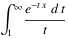## Solution to Integral of the Week #5

January 22nd, 2009 | Categories: general math, Problem of the week | Tags:

Near the beginning of the month I posted a new Integral of the week which asked for the indefinite integral of

W(1/x)

Where W is the Lambert W function.  I had a response from Simon Tyler who sent me a PDF file with a full solution and as far as I can tell it is correct but feel free to get in touch if you disagree with us.

In case you don’t want to read the PDF file, the solution is

x*W(1/x) + E1(W(1/z))

Where E1 stands for the Exponential Integral which is defined asIn Mathematica notation this solution is written as

x*ProductLog[1/x] + ExpIntegralE[1, ProductLog[1/x]]

Since Mathematica uses the function name ProductLog to stand for the Lambert W function.  Let’s differentiate this to see if it is correct

D[x*ProductLog[1/x] + ExpIntegralE[1, ProductLog[1/x]], x]

gives ProductLog[1/x]

So, why did I select this particular integral as integral of the week you may ask?  Well it started off with a thread in the Maxima developers mailing list.  The developers of Maxima added the Lambert W function fairly recently and the system was having serious problems finding the integral W(1/x).  If you evaluate

integrate(lambert_w(1/x),x)

in Maxima 5.17.1 then the integrator just loops endlessly – never finding a solution. The members of the maxima developers forum knew what the result should be but they couldn’t get maxima to find it. This got me curious so I fired up Mathematica 7 and did

Integrate[ProductLog[1/x],x]

Mathematica replied by simply returning the unevaluated integral to me. So, not only did Maxima fail at this particular integral but so did Mathematica. Could MATLAB 2008b do any better with its new Mupad-based symbolic toolbox?
``` syms x int(lambertw(0, 1/x), x)```

Warning: Explicit integral could not be found.
> In sym.int at 64

That’ll be a no then! I can’t try Maple 12 since I don’t have a copy but I do have a copy of Matlab 2007b which uses the Maple 10 kernel as part of its symbolic toolbox so how did that do?
``` syms x int(lambertw(0, 1/x), x)```

ans=x*lambertw(1/x)+Ei(1,lambertw(1/x))

Success! So Maple (via MATLAB 2007b) is the winner in this little game it seems – I really need to get myself a full copy of the thing.

So, since 3 out of 4 powerful computer algebra systems couldn’t do this integral, I wondered if maybe I could. Turns out that I couldn’t! It seems my interest in bizarre looking integrals far outstrips my ability to actually do them. So, I threw it open to you guys and, thankfully, Simon rose to the challenge. Thanks again Simon.